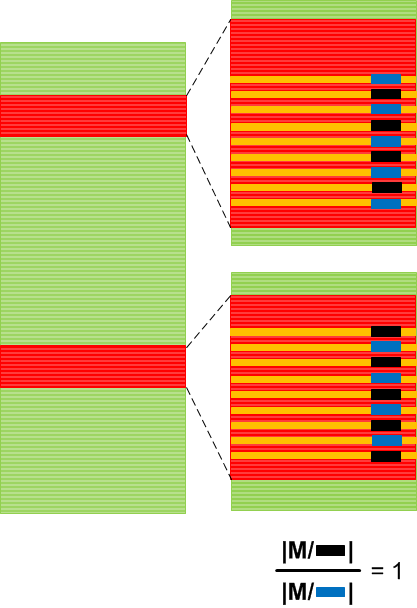## Trace Analysis Patterns (Part 78)

The next trace and log analysis pattern name is borrowed from Factor Groups in mathematics (or quotient groups). Here a group is, of course, not a mathematical group but just a group (or set) of log messages or trace statements. However, every trace message has variable and invariant parts. Variable parts usually contain some values, addresses or status bits. It can even be string values. Such values form a set too and can be partitioned into disjoint (non-overlapping) subsets. For example, a window foreground status can be either true or false. And we can group messages into disjoint factor groups each one having either only true or only false foreground status. The following trace graph illustrates a WindowHistory64 log where it was reported that one window was periodically loosing and gaining focus:We found messages related to the reported process window title. By using Density Distribution pattern another such group of messages was found for another process window. Then a factor group was formed with two subgroups and their Relative Density was compared. For correlated alternating values it was expected to be 1. This was a very simple case, of course, which was analysed just by looking at a textual log but in more complex cases a computer assistance is required. A member of a factor group can also be generalized as a message subset with messages having variable part values from some domain subset or even calculated from it (some sort of a predicate): Mi = { m | P(m) }, where the original group of messages is a disjoin union of such message subsets: M = U Mi.

- Dmitry Vostokov @ DumpAnalysis.org + TraceAnalysis.org -

You must be logged in to post a comment.